## College Physics

Physics & Astronomy

## Quiz 3 : Current and ResistanceLooking for Introductory Physics Homework Help?# Quiz 3 : Current and Resistance

In case of electrostatics, the total charge resides on the surface of a conductor. This means that the charge inside the conductor is zero. So, the electric field inside the conductor is always zero. In case of current carrying conductor, the current arises because of a potential difference applied between the ends of the conductor. This produces a nonzero internal electric field.

(a) Current is the rate of flow of charge.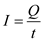Here,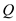is the charge and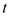is the time. Charge is the integral multiple of charge of electron.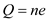Here, n is an integer,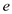is the charge of electron. Substitutein the equation.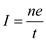Rearrange the equationfor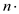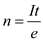Convert the units of current from mA to A.Convert the unit of time min to s.Substitute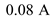for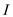,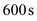forand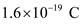forin the equation.Therefore, number of electrons flows through the metal wire is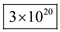. (b) Since electrons have a negative charge and their direction is opposite to the direction of current.

The atomic vibrations in matter are increased with increasing temperature. These vibrating atoms collide with the electrons which were flowing through the wire. If the vibrations are more, then the collisions are also more. Thus, the current flow in the wire decreases. That is the resistance of the wire as well as resistivity of the wire is increased with increasing temperature.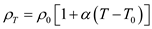Here,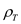is the resistivity of the metal at temperature T ,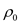is the resistivity of the metal at temperature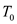, and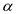is the temperature coefficient of the resistivity Therefore, the resistivity of metals increases with temperature according to following equation.